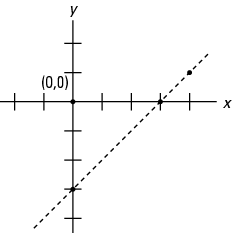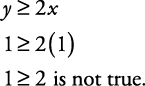## Linear Inequalities and Half-Planes

Each line plotted on a coordinate graph divides the graph (or plane) into two half‐planes. This line is called the boundary line (or bounding line). The graph of a linear inequality is always a half‐plane. Before graphing a linear inequality, you must first find or use the equation of the line to make a boundary line.

#### Open half-plane

If the inequality is a “>” or “<”, then the graph will be an open half‐plane. An open half‐plane does not include the boundary line, so the boundary line is written as a dashed line on the graph.

##### Example 1

Graph the inequality y < x – 3.

First graph the line y = x – 3 to find the boundary line (use a dashed line, since the inequality is “<”) as shown in Figure 1.

Figure 1. Graph of boundary line for y < x – 3.x

y

3

0

0

-3

4

1

Now shade the lower half‐plane as shown in Figure 2, since y < x – 3.

Figure 2. Graph of inequality y < x – 3.To check to see whether you've shaded the correct half‐plane, plug in a pair of coordinates—the pair of (0, 0) is often a good choice. If the coordinates you selected make the inequality a true statement when plugged in, then you should shade the half‐plane containing those coordinates. If the coordinates you selected do not make the inequality a true statement, then shade the half‐plane not containing those coordinates.

Since the point (0, 0) does not make this inequality a true statement,

y < x – 3

0 < 0 – 3 is not true.

You should shade the side that does not contain the point (0, 0).

This checking method is often simply used as the method to decide which half‐plane to shade.

#### Closed half-plane

If the inequality is a “≤”or “≥”, then the graph will be a closed half‐plane. A closed half‐plane includes the boundary line and is graphed using a solid line and shading.

##### Example 2

Graph the inequality 2 xy ≤ 0.

First transform the inequality so that y is the left member.

Subtracting 2 x from each side gives

y ≤ –2 x

Now dividing each side by –1 (and changing the direction of the inequality) gives

y ≥ 2 x

Graph y = 2 x to find the boundary (use a solid line, because the inequality is “≥”) as shown in Figure 3.

Figure 3. Graph of the boundary line for y ≥ 2x.x

y

0

0

1

2

2

4

Since y ≥ 2 x, you should shade the upper half‐plane. If in doubt, or to check, plug in a pair of coordinates. Try the pair (1, 1).So you should shade the half‐plane that does not contain (1, 1) as shown in Figure 4.

Figure 4. Graph of inequality y ≥ 2 x.Back to Top
A18ACD436D5A3997E3DA2573E3FD792A